Title: Two Years of Bayesian Bandits for E-Commerce

Abstract: At Monetate, we've deployed Bayesian bandits (both noncontextual and contextual) to help our clients optimize their e-commerce sites since early 2016. This talk is an overview of the lessons we've learned from both the processes of deploying real-time Bayesian machine learning systems at scale and building a data product on top of these systems that is accessible to non-technical users (marketers). This talk will cover

• the place of multi-armed bandits in the A/B testing industry,
• Thompson sampling and the basic theory of Bayesian bandits,
• Bayesian approaches for accommodating nonstationarity in bandit feedback,
• user experience challenges in driving adoption of these technologies by nontechnical marketers.

We will focus primarily on noncontextual bandits and give a brief overview of these problems in the contextual setting as time permits.

Bio: Austin Rochford is Chief Data Scientist at Monetate, where he does research and development for machine learning-driven marketing products. He is a recovering mathematician, a passionate Bayesian, and a PyMC3 developer.

A Dockerfile that will produce a container with the dependenceis of this notebook is available here.

In :
%matplotlib inline

In :
from tqdm import tqdm, trange

In :
from matplotlib import pyplot as plt
from matplotlib.dates import DateFormatter, MonthLocator
from matplotlib.ticker import StrMethodFormatter
import numpy as np
import pandas as pd
import scipy as sp
import seaborn as sns

In :
SEED = 76224 # from random.org, for reproducibiliy
np.random.seed(SEED)

In :
sns.set()
C = sns.color_palette()

pct_formatter = StrMethodFormatter('{x:.1%}')

#configure matplotlib
FIGURE_WIDTH = 8
FIGURE_HEIGHT = 6
plt.rc('figure', figsize=(FIGURE_WIDTH, FIGURE_HEIGHT))

LABELSIZE = 14
plt.rc('axes', labelsize=LABELSIZE)
plt.rc('axes', titlesize=LABELSIZE)
plt.rc('figure', titlesize=LABELSIZE)
plt.rc('legend', fontsize=LABELSIZE)
plt.rc('xtick', labelsize=LABELSIZE)
plt.rc('ytick', labelsize=LABELSIZE)


# Two Years of Bayesian Bandits for E-Commerce¶## NYC College of Technology • April, 18 2019 • @AustinRochford¶#### [email protected] • [email protected]¶• Founded 2008, web optimization and personalization SaaS
• Observed 5B impressions and \$4.1B in revenue during Cyber Week 2017

#### Non-technical marketer-focused¶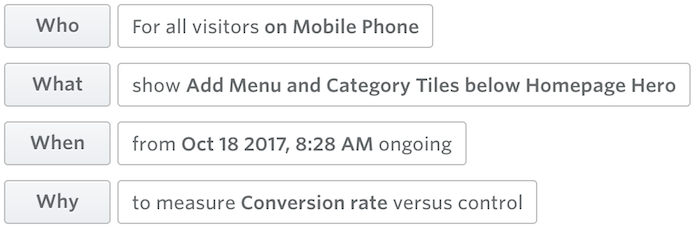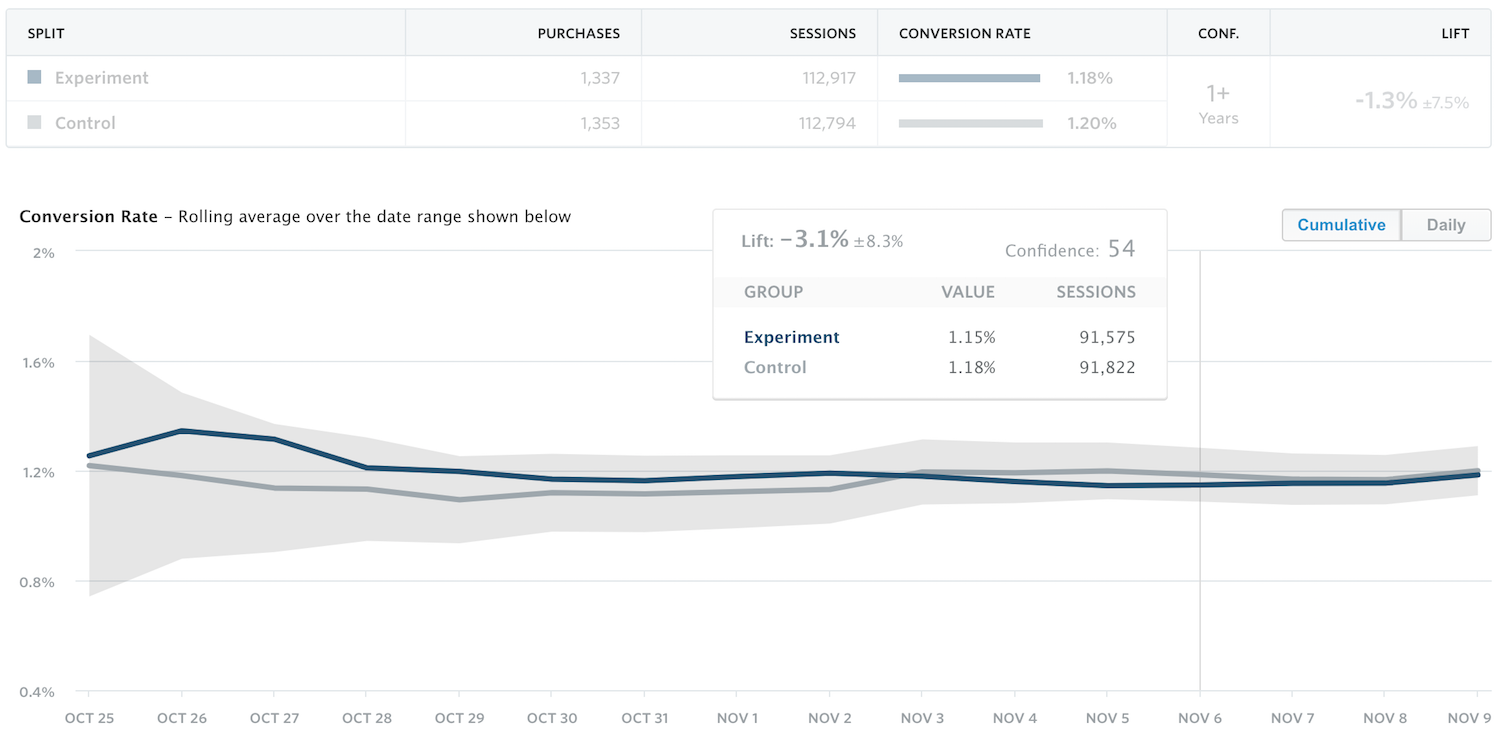### Outline¶

• Web optimization
• A/B testing
• Multi-armed bandits
• Bayesian bandits
• Thompson sampling
• Bandit bias
• Inverse propensity weighting

## Web Optimization¶

### A/B testing¶#### A/B testing machinery¶Ronald FisherWhom the Gods Would Destroy, They First Give Real-time Analytics

#### Sequential testing¶Abraham Wald

#### Sequential optimization¶Much of the A/B testing industry uses classical Fisher/Neyman-Pearson style fixed-horizon frequentist significance tests. Sophistocated approaches use sequential hypothesis testing. We've found, through many years of interaction with marketers, that they take a fundamentally Bayesian view of the world. Most interpret p-values as the "probability that the experiment is better/worse than the control."

### Multi-armed bandits¶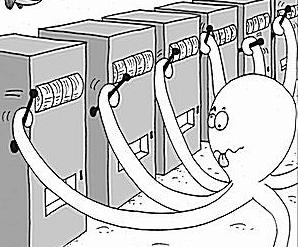Multi-armed bandit problems are extensively studied and come in many variantions. Here we focus on the simplest multi-armed bandit objective, regret minimization.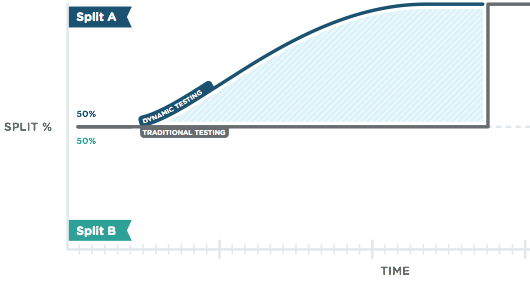#### Multi-armed bandit systems¶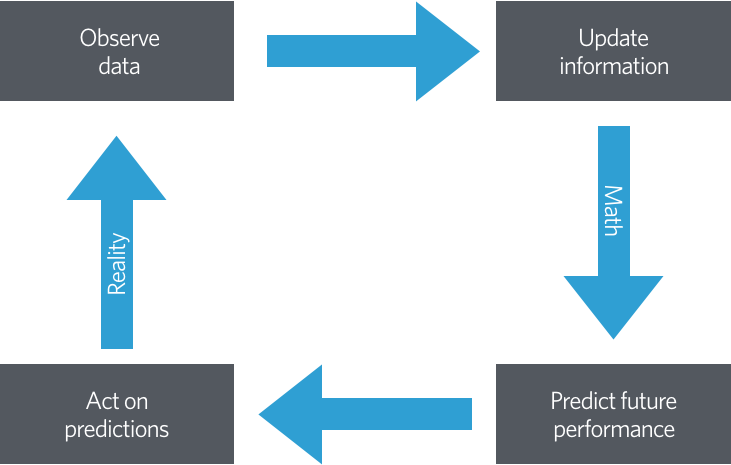## Bayesian Bandits¶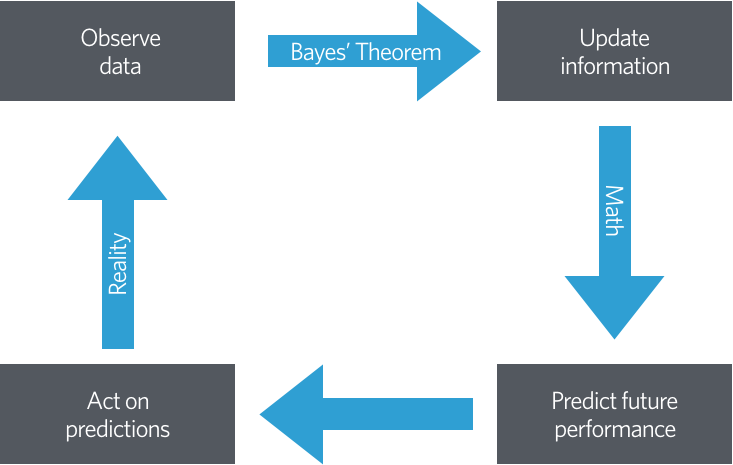### Beta-binomial model¶

\begin{align*} x_A, x_B & = \textrm{number of rewards from users shown variant } A, B \\ x_A & \sim \textrm{Binomial}(n_A, r_A) \\ x_B & \sim \textrm{Binomial}(n_B, r_B) \\ r_A, r_B & \sim \textrm{Beta}(1, 1) \end{align*}
\begin{align*} r_A\ |\ n_A, x_A & \sim \textrm{Beta}(x_A + 1, n_A - x_A + 1) \\ r_B\ |\ n_B, x_B & \sim \textrm{Beta}(x_B + 1, n_B - x_B + 1) \end{align*}

### Thompson sampling¶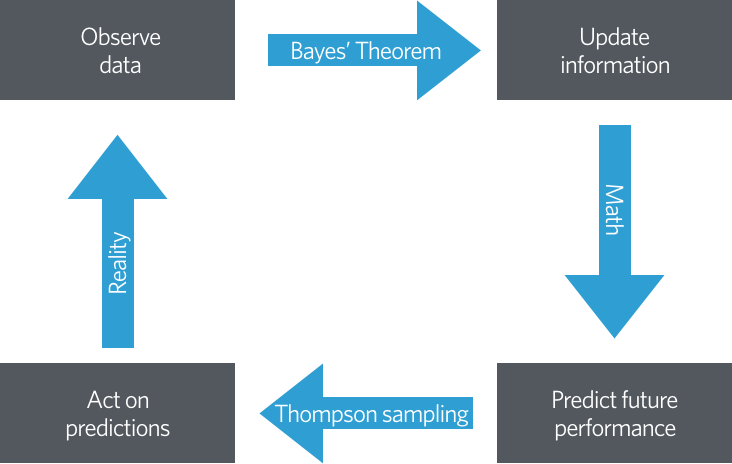Thompson sampling randomizes user/variant assignment according to the probabilty that each variant maximizes the posterior expected reward.

The probability that a user is assigned variant A is

\begin{align*} P(r_A > r_B\ |\ \mathcal{D}) & = \int_0^1 P(r_A > r\ |\ \mathcal{D})\ \pi_B(r\ |\ \mathcal{D})\ dr \\ & = \int_0^1 \left(\int_r^1 \pi_A(s\ |\ \mathcal{D})\ ds\right)\ \pi_B(r\ |\ \mathcal{D})\ dr \\ & \propto \int_0^1 \left(\int_r^1 s^{\alpha_A - 1} (1 - s)^{\beta_A - 1}\ ds\right) r^{\alpha_B - 1} (1 - r)^{\beta_B - 1}\ dr \end{align*}

#### Monte Carlo Methods¶

In :
N = 5000

x, y = np.random.uniform(0, 1, size=(2, N))

In :
fig, ax = plt.subplots()
ax.set_aspect('equal');

ax.scatter(x, y, c='k', alpha=0.5);

ax.set_xticks([0, 1]);
ax.set_xlim(0, 1.01);

ax.set_yticks([0, 1]);
ax.set_ylim(0, 1.01);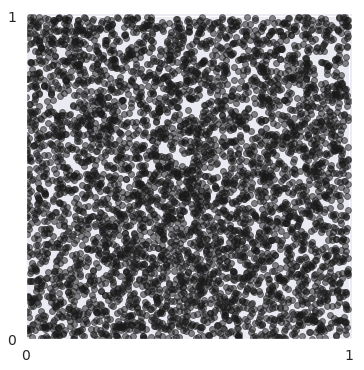In :
fig

Out: